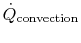Thermodynamics and Propulsion

# 19.5 Muddiest Points on Chapter 19

MP 19..1   Which bodies does the radiation heat transfer occur between in the thermocouple?

The radiation heat transfer was between the walls (or more generally the boundaries of the duct) and the thermocouple. The boundaries may not be at the same temperature of the flowing fluid, for example in a turbine. If the boundaries are not at the same temperature as the fluid, and they radiate to the thermocouple, there can be an error in the temperature that the thermocouple reads. The discussion was about different possible sources of this type of error.

MP 19..2   Still muddy about thermocouples.

I didn't mean to strew confusion about these. As in the notes, if we take a pair of dissimilar wires, say copper and constantan (an alloy of tin and several other metals), or platinum and rhodium, which are joined at both ends and subject the two junctions to a temperature difference, it is found that a voltage difference will be created. If we know the temperature of one junction (say by use of an ice bath) and we know the relation between voltage and temperature difference (these have been measured in detail) we can find the temperature of the other junction from measurement of the voltage. The assumption is that the temperature of the junction is the temperature that is of interest.

MP 19..3   Why does increasing the local flow velocity decrease the temperature error for the thermocouple?

The heat transferred by convection is). If we neglect conduction from the wire junction (in other words assume the thermocouple wires are thin and long), the heat balance is between convection heat transfer and radiation heat transfer. For a fixedif the heat transfer increases the temperature difference between the thermocouple and the fluid decreases. We have seen, however, that the heat transfer may be estimated using the Reynolds analogy (Section 17.1). For fixed skin friction coefficient the higher the velocity the higher the heat transfer coefficient.

UnifiedTP